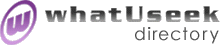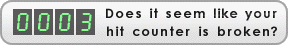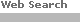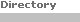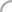SearchThe Directory The Webfor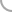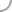Java_AppletsNew! Submit a site

whatUseek Collection Sites (submit a site ):

Give your site great placement in this category in as little as two business days!

whatUseek Directory Site Listings:

Chaos and Fractals Applets - Several java applets for use in exploring the topics of chaos and fractals.

Euclid's Elements - Joyce's Java version - This dynamically illustrated edition of Euclid's Elements includes 13 books on plane geometry, geometric and abstract algebra, number theory, incommensurables, and solid geometry.

Experimental Math Applets - Some applets covering Besicovitch sets, conformal compactifaction, honeycombs, exponent calculator, the complex plane, elementary complex maps, Möbius transforms, multi-valued functions, the complex derivative, the complex integral, Taylor and Laurent expansions.

Famous Curves - About 60 famous mathematical graphs or curves drawn by applets.

Graph Explorer - A Java applet for graphing functions, with smooth zooming and panning across graphs, and variable parameters which can be used for animation.

Graph It ! - The power of Javascript - This applet uses dynamically created pictures to draw the graph of your mathematical function. It is a good example of the possibilities of Javascript.

HKCEE Mathematics - This web page contains 7 Java Applets for exploring Geometry and Trigonometry.

Java Applets for Visualization of Statistical Concepts - These applets are designed for the purpose of computer-aided education in statistic courses. The intent of these applets is to help students learn some abstract statistics concepts easier than before. The approach taken here is based upon visualization and simulation.

Java Demos for Probability and Statistics - College professor's applets.

Manipula Math with Java - Java applets demonstrating many geometrical and other theorems

Math Java Applets - About 15 applets covering a number of math problems and principles.

Online Tutor - on-line math tutor and science tutor for school age children - Place values to Probability, geometry, ratios, percentages, fractions and measurements, solar system, weather and human body

Spirograph Applet - Makes a spirograph, just like the kid toy.

TenBlocks and IntegerZone - TenBlocks turns the times tables into a series of puzzles. IntegerZone lets users explore aspects of arithmetic and number theory using the integers themselves as the interface.

xFunctions - The xFunctions applet covers several aspects of calculus and pre-calculus mathematics, including graphs, parametric curves, derivatives, Riemann sums, and integral curves.Help build the largest human-edited directory on the web.About   Help   Content Filter   Terms   Privacy Policy© 2018 whatUseek